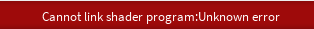#### Howdy, Stranger!

We are about to switch to a new forum software. Until then we have removed the registration on this forum.

# sending array to Shader, unexpected error

edited June 2016

So I am trying to get a simple particle system to work with shaders.

my processing code looks like this

``````PShader pointShader;
int COUNT = 300;

float[] sourceX = new float[COUNT];
float[] sourceY = new float[COUNT];

void setup()
{
size(600, 600, P3D);
background(255);

}

void draw() {
// background(0);
for (int i = 0; i < COUNT; i++) {
sourceX[i] = random(0, width);
sourceY[i] = random(0, height);
}

rect(0, 0, width, height);
//noLoop();

}
``````

``````#define PROCESSING_COLOR_SHADER

#ifdef GL_ES
precision mediump float;
precision mediump int;
#endif

uniform float sourceX;
uniform float sourceY;

void main() {
float intensity = 0.0;

// sum the distances from the points
for(int i = 0; i < 300; i++) {
float d = distance(gl_FragCoord.xy, vec2(sourceX[i], sourceY[i]));
intensity += exp(-0.05 * d * d);
}

// map the colours

gl_FragColor = vec4(0.0, intensity * 1, 1.0, 1.0);

}
``````

this works without a problem but as soon as I up the particle count to 1000 I get the error:Where am I going wrong here? Is this not the right way to do this?

Ultimately my intention is to send the point cloud data from a Kinect2 to the shader. I have a working sketch for that (without the shader) but instead of sending the Kinect points into a class I would like to send it to a shader. I'm hoping I can have a much higher particle count that way.

Tagged:

• Are you sure the error is array related? Try commenting out bits until the error goes away.

I am suspicious of line 5 but can't test anything here / now.

(Oh, sorry, didn't see the bit about the 1000 particles. That does seem odd)

• I don't have the solution, but only questions :) Does this produce 1000 iterations for every single pixel on the screen? Is it slower when you increase the number of particles? Would the effect look very different if you had 1000 quads with a radial texture applied to them, and maybe using blend mode ADD?

• ``````    float d = distance(gl_FragCoord.xy, vec2(sourceX[i], sourceY[i]));
intensity += exp(-0.05 * d * d);
``````

you could get rid of the 300 expensive sqrts per fragment by not calling distance() - you square the result again immediately so why bother?

something like

``````float d2 = myDistance2(gl_FragCoord.xy, vec2(sourceX[i], sourceY[i]));
intensity += exp(-.05 * d2);
``````

where myDistance2 is something like

``````myDistance2(vec2 a, vec2 b) {
return (a.x - b.x) * (a.x - b.x) + (a.y - b.y) * (a.y - b.y);
}
``````

(massively untested)